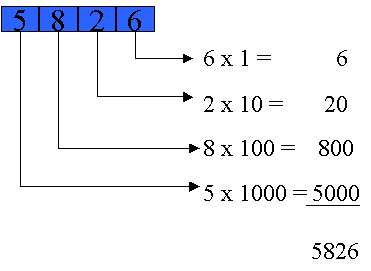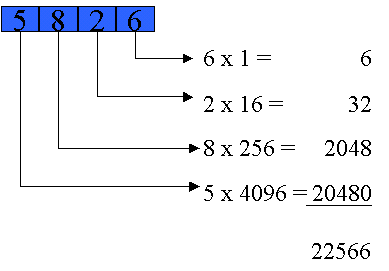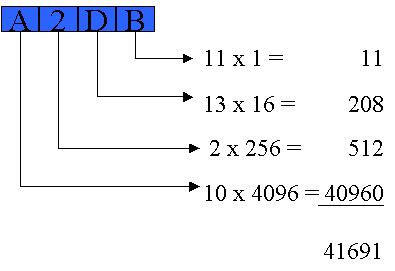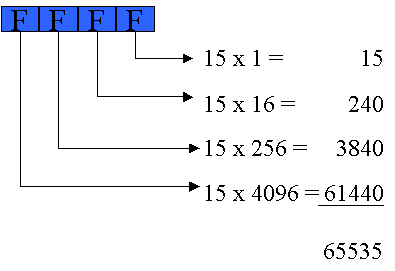The hexadecimal (base 16) number system operates the same way as the decimal (base 10) number system, except it is based on sixteen instead of ten. The operation of the decimal system is familiar.

The 4-digit base-10 number 5826 appears below, indicating how the value of the number is derived from the values of its 4 digits.Everybody knows that 5826 means five-thousand eight-hundred twenty-six. But only because they have been taught that 1, 10, 100, and 1000 are part of the calculation even though they are never written. All that is actually written is a total of twenty-one (5 and 8 and 2 and 6). Only the interpretation that people supply, which is purely mental and unwritten, informs what is written with its intended value.

Hexadecimal operates the same way. Each digit is "weighted" by a "multiplier," with the results all added together. The multipliers in both systems are the powers of the system base (10 or 16). The powers of 10 are 1, 10, 100, 1000, etc. while those of 16 are 1, 16, 256, 4096, etc. So the same digits "5826" used in base 16 represent a value calculated as follows:Though the digits are the same (5826) the values come out quite different, because the base and its "multiplier values" are different.

You will see hexadecimal numbers some of whose digits are letters instead of numbers. That's because the number of digits needed by any number system is the number's base. So base base 2 needs 2 digits, base 10 needs 10,  and base 16 needs 16. Base 2 has 0 and 1. Base 10 has 0 through 9. Base 16 borrows 0 though 9 but needs another 6. For those, we could invent some symbols. However, for convenience we employ the first 6 letters of the alphabet (A through F) instead. When we run out of digits at 9, we use A as the next digit. So A represents the value 10. B comes next, and represents 11. The hexadecimal digits and thevalue they stand for are:

 Hexadecimal digit Value 0 0 1 1 2 2 3 3 4 4 5 5 6 6 7 7 8 8 9 9 A 10 B 11 C 12 D 13 E 14 F 15

Here's the derivation of the value of another 4-digit hexadecimal number, but this one uses some of the high-order digits A-F:The highest you can count with a given number of digits (in any number system) is the number in which every digit contains the maximum value in the number system (1 in base 2, or 9 in base 10, or F in base 16). So the largest number you can represent with 4 digits in base 16 is:Counting any higher than that would require that you utilize more digits. The very next number is 10000 in hexadecimal, or 65536 in decimal. It is a well-known value in computer science and is called "64K."